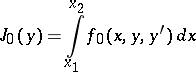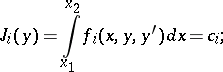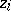# Isoperimetric problem

One of the fundamental problems in the classical calculus of variations. The isoperimetric problem consists in minimizing a functionalunder constraints of the formand certain boundary conditions.

The isoperimetric problem reduces to the Lagrange problem when new variablesare introduced satisfying the differential equationswith boundary conditionsNecessary conditions for optimality in the isoperimetric problem have the same form as do the simplest problems in the calculus of variations related to the Lagrange functionThe name "isoperimetric problem" goes back to the following classical question: Among all the curves with given perimeter in the plane, find the one that bounds the largest area.